This is the note I used as an example of applications in Linear Algebra I lectured at Purdue University. It is slightly modified so that it is more or less self contained.

Principal Component Analysis (PCA) is a linear algebra technique for data analysis, which is an application of eigenvalues and eigenvectors. PCA can be used in

1. exploratory data analysis (visualizing the data)
2. features reduction

We will learn the basic idea of PCA and see its applications in handwritten-digits recognition, eigenfaces and etc.

import numpy as np
import sklearn.linear_model
import matplotlib.pyplot as plt
from PIL import Image
import tarfile
from IPython.display import set_matplotlib_formats

plt.rcParams["figure.figsize"] = (8, 6)
set_matplotlib_formats('png', 'pdf')


## Intuition

Let $\mathcal{B} = \{v_1, \dots, v_n\}$ be an ordered basis of $\mathbb{R}^n$. Let $x \in \mathbb{R}^n$ be a vector such that

$$x = x_1^{\prime}v_1 + \cdots + x_n^{\prime}v_n,$$

then

$$x_{\mathcal{B}} = \begin{bmatrix} x_1^{\prime} \\ \vdots \\ x_n^{\prime} \end{bmatrix}$$

is called the $\mathcal{B}$-coordinate of $x$. Most of the time, we just use the standard basis and the standard coordinate system. However, in certain cases, using an ordered basis can bring more insights of a given dateset.

Example. We generate a fake dataset and see that an ordered basis is a better choice to view this fake dataset.

# generate and plot the fake dataset
x = np.arange(-4, 4)
y = x + np.random.randn(len(x))
plt.plot(x, y, 'ro')
x = np.linspace(-4, 4, 100)
plt.plot(x, x, 'b')

plt.xticks([])
plt.yticks([])
ax = plt.gca()
ax.spines['right'].set_color('none')
ax.spines['top'].set_color('none')
ax.xaxis.set_ticks_position('bottom')
ax.spines['bottom'].set_position(('data', 0))
ax.yaxis.set_ticks_position('left')
ax.spines['left'].set_position(('data', 0))

plt.show()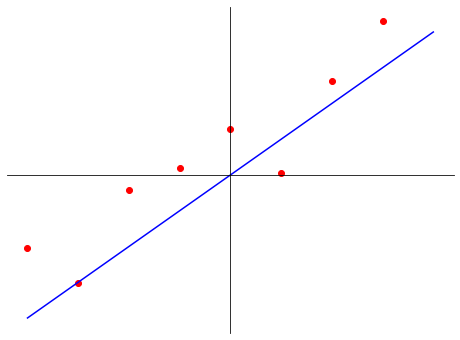In this fake dataset, all data points are close to the blue line. If we use this blue line and another line that is orthogonal to it to form a new coordinate system, these data points have the second components more or less zeros. In other words, the first (principal) componet in the new coordinate system captures most of the information for this dataset.

Question. Given a set of data $\{x_1, x_2, ..., x_p\}$, each of $x_i \in \mathbb{R}^n$, how can we find the first principal axis? That is, how can we find the direction of the axis that captures most the information of the dataset.

Suppose the first principal axis is represented by a unit vector $u$, then the first principal component of $x_i$ is the projection of $x_i$ on $u$:

$$\frac{x_i \cdot u}{u \cdot u} = x_i \cdot u.$$

We want to find a unit vector $u \in \mathbb{R}^n$ such that $\frac{1}{p}\sum_{i = 1}^p (x_i \cdot u)^2$ is maximized. Recall that the dot product and matrix multiplication are related:

$$x_i \cdot u = x_i^t u = u^t x_i.$$

Then

$$(x_i \cdot u)^2 = u^t x_i x_i^t u = u^t (x_ix_i^t) u.$$

If we set $X = \frac{1}{p}\sum_{i=1}^p x_ix_i^t$, then we want to find a unit vector $u$ to maximize

$$\frac{1}{p}\sum_{i = 1}^p (x_i \cdot u)^2 = \frac{1}{p}\sum_{i = 1}^p u^t (x_ix_i^t) u = u^t \left(\frac{1}{p}\sum_{i = 1}^p x_ix_i^t \right) u = u^t X u.$$

Answer. $u$ is an eigenvector of $X$ with the largest eigenvalues. More generally, the first $k$ components are given by eigenvectos of the first $k$ largest eigenvalues of $X$.

## Algorithm

Suppose we have a set of data $\{x_1, x_2, ..., x_p\}$, each of $x_i \in \mathbb{R}^n$. We divide the algorithm of PCA into 3 steps.

Step 1. Normalize data to zero mean and unit variance.

Normalization to zero mean is necessary for the correctness of the algorithm.

x = np.arange(-4, 4)
y = x + np.random.randn(len(x))
plt.plot(x, y, 'ro', label="zero mean data")
z = np.linspace(-4, 4, 100)
plt.plot(z, z, 'r')

plt.plot(x, y - 3, 'bo', label="true data")
plt.plot(z, z - 3, 'b')

plt.xticks([])
plt.yticks([])
ax = plt.gca()
ax.spines['right'].set_color('none')
ax.spines['top'].set_color('none')
ax.xaxis.set_ticks_position('bottom')
ax.spines['bottom'].set_position(('data', 0))
ax.yaxis.set_ticks_position('left')
ax.spines['left'].set_position(('data', 0))

plt.legend()
plt.show()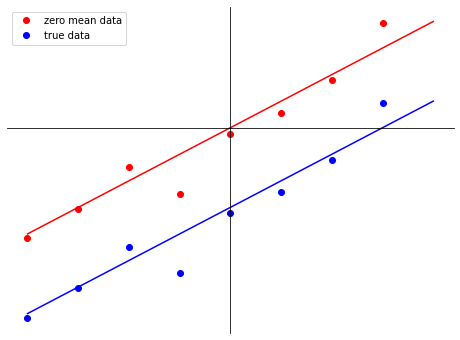We use the above generated figure to explain the intuition of normalization to zero mean. The blue dots represent the dataset we have, and the blue line represent the desired "first principal axis". However, to represent the blue line, we need to know not only the direction of line, but also a point on the line. We could choose a point together with the direction to represent the blue line, but it is better to shift the blue data to the red ones so that the desired axis passing through the origin. Then we just need to keep track of the direction, for which we can compute as an eigenvector of a certain matrix.

Normalization to unit variance is to eliminate the discrepency of different scales of components. If we are sure that all components are of the same scale, then we can skip this part of normalization.

Zero mean:

• find the mean $\mu = \frac{1}{p} \sum_{i = 1}^{p} x_i$
• replace $x_i$ by $x_i - \mu$

Unit variance ($x_{i, j}$ is the $j$-th component of $x_i$):

• find the variance $\sigma_j^2 = \frac{1}{p} \sum_{i = 1}^p x_{i, j}^2$
• replace $x_{i, j}$ by $\frac{x_{i, j}}{\sigma_j}$

Step 2. Compute $X = \frac{1}{p} \sum_{i = 1}^p x_i x_i^t$. This matrix $X$ is called the covariance matrix of the normalized data.

Step 3. Find all unit eigenvectors of $X$, ordered by eigenvalues in descending order.

Example. Suppose we have a set of data

$$\left\{ \begin{bmatrix} 5 \\ 4 \end{bmatrix}, \begin{bmatrix} 10 \\ 11 \end{bmatrix}, \begin{bmatrix} 12 \\ 12 \end{bmatrix} \right\}$$

We assume that the data are of the same scale. Find the first principal component of a data point $y = \begin{bmatrix} 8 \\ 6 \end{bmatrix}$.

Step 1. Normalization to zero mean.

$$\mu = \frac{1}{3} \left( \begin{bmatrix} 5 \\ 4 \end{bmatrix} + \begin{bmatrix} 10 \\ 11 \end{bmatrix} + \begin{bmatrix} 12 \\ 12 \end{bmatrix} \right) = \begin{bmatrix} 9 \\ 9 \end{bmatrix},$$

so the normalized data is

$$\left\{ \begin{bmatrix} -4 \\ -5 \end{bmatrix}, \begin{bmatrix} 1 \\ 2 \end{bmatrix}, \begin{bmatrix} 3 \\ 3 \end{bmatrix} \right\}$$

Since the data are of the same scale by assumption, we don't need to do variance normailization.

Step 2. Compute the covariance matrix.

\begin{align*} X &= \frac{1}{3} \left( \begin{bmatrix} -4 \\ -5 \end{bmatrix} \begin{bmatrix} -4 & -5 \end{bmatrix} + \begin{bmatrix} 1 \\ 2 \end{bmatrix} \begin{bmatrix} 1 & 2 \end{bmatrix} + \begin{bmatrix} 3 \\ 3 \end{bmatrix} \begin{bmatrix} 3 & 3 \end{bmatrix} \right) \\ &= \frac{1}{3} \begin{bmatrix} -4 & 1 & 3 \\ -5 & 2 & 3 \end{bmatrix} \begin{bmatrix} -4 & -5 \\ 1 & 2 \\ 3 & 3 \end{bmatrix} \\ &= \begin{bmatrix} \frac{26}{3} & \frac{31}{3} \\ \frac{31}{3} & \frac{38}{3}\end{bmatrix} \end{align*}

Step 3. Find all unit eigenvectors of $X$.

$X$ has eigenvalue $\lambda \approx 21.19$ with eigenvector

$$u_1 = \begin{bmatrix} 0.64 \\ 0.77 \end{bmatrix}$$

and eigenvalue $\lambda \approx 0.14$ with eigenvector

$$u_2 = \begin{bmatrix} 0.77 \\ -0.64 \end{bmatrix}$$

Finally, we find the first principal component of $y = \begin{bmatrix} 8 \\ 6 \end{bmatrix}$. We normalize $y$ by the mean of the data

$$\bar{y} = y - \mu = \begin{bmatrix} -1 \\ -3\end{bmatrix}.$$

Then the first principal component is

$$\bar{y} \cdot u_1 = -0.64 - 3 (0.77) = -2.95.$$

Remark. For each principal axis, there are two possible choices of directions, $u$ and $-u$. Both choices are good, since they will only change the signs of the projections on the axis.

## MNIST handwritten-digits dataset

As an example, we are going to do a PCA analysis on the MNIST dataset (http://yann.lecun.com/exdb/mnist/)

with open('train-labels.idx1-ubyte', 'rb') as lbpath:
mnist_labels = np.frombuffer(lbpath.read(), dtype=np.uint8, offset=8)
with open('train-images.idx3-ubyte', 'rb') as imgpath:
mnist_images = np.frombuffer(imgpath.read(), dtype=np.uint8,
offset=16).reshape(-1, 784)

img = mnist_images.reshape((28, 28))
plt.imshow(img)
plt.axis("off")
plt.colorbar()
plt.show()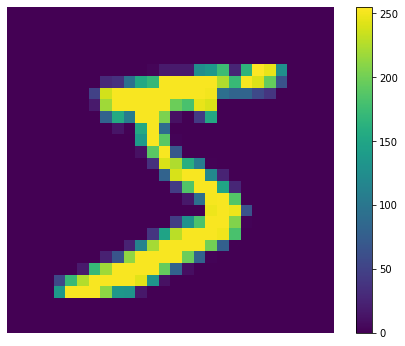# Step 1: normalization to zero mean
mnist_mean = np.mean(mnist_images, axis=0)
normalized_mnist_images = mnist_images - mnist_mean

img = normalized_mnist_images.reshape((28, 28))
plt.imshow(img)
plt.axis("off")
plt.colorbar()
plt.show()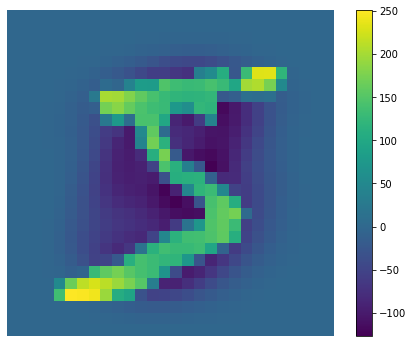# Step 2: compute the covariance matrix
# note: we put an image as a row vector in normalized_mnist_images
# instead of a column vector. So the formula to find the covariance
# matrix is a little bit different than the one given above

X = normalized_mnist_images.transpose() @ normalized_mnist_images
X /= normalized_mnist_images.size
X.shape

(784, 784)
# Step 3: find the eigenvalues and eigenvectors
# we sort the eigenvalues in descending order

# X is symmetric and its eigenvalues are real
mnist_values, mnist_vectors = map(np.real, np.linalg.eig(X))
idx = np.argsort(mnist_values)[::-1]
mnist_values = mnist_values[idx]
mnist_vectors = mnist_vectors[:, idx]


Since eigenvetors are in the same ambient space of the images, we can treat an eigenvector as an image. Let's take a look at the first three eigenvectors.

k = 3
plt.figure(figsize=(12, 3))
for i in range(k):
plt.subplot(1, k, i + 1)
img = mnist_vectors[:, i]
img = img.reshape((28, 28))
plt.imshow(img)
plt.axis("off")
plt.colorbar()
plt.show()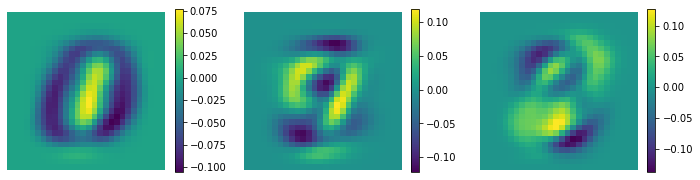For each picture of the eigenvectors, we should focus on the values that are far from 0. That is, we should search for purple and yellow areas in the figure above. In the first example, purple and yellow areas concentrate on the center of the picture, with purple area like a 0 and yellow area like an 1. That makes a lot of sense, because the handwritten digits are in the center of the picture, and this particular eigenvector might try to capture 0 and 1. In fact, all eigenvectors shown in the figure above has "hot area" in the center, only with different patterns. This is an indication that PCA can indeed capture useful informations of a dataset.

Next, let's compute the first 3 principal component of the first normalized image (digit 5).

# we take the first normalized image, not the original image
img = normalized_mnist_images
components = [
np.dot(img, mnist_vectors[:, i]) for i in range(k)
]
components

[-123.93258865866045, -312.67426202272094, -24.514051755586404]
img_proj = sum(components[i] * mnist_vectors[:, i] for i in range(k))
plt.imshow(img_proj.reshape((28, 28)))
plt.axis("off")
plt.colorbar()
plt.show()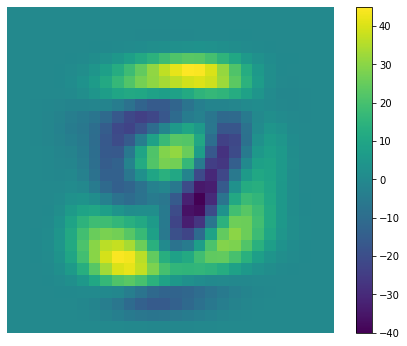We don't see the digit 5 in the plot above because $k = 3$ is too small, and the first 3 principal components is not enough to reconstruct the digit 5. As we increase the value $k$, we can reconstruct a better approximation of the original image.

num_of_components = [5, 10, 30, 50, 100, 200]
n = len(num_of_components)
plt.figure(figsize=(12, 3))
plt.subplot(1, n + 1, 1)
plt.imshow(img.reshape(28, 28))
plt.axis('off')
plt.title("original image")

components = [
np.dot(img, mnist_vectors[:, i])
for i in range(num_of_components[-1])
]
for j, k in enumerate(num_of_components):
plt.subplot(1, n + 1, j + 2)
img_proj = sum(components[i] * mnist_vectors[:, i] for i in range(k))
plt.imshow(img_proj.reshape((28, 28)))
plt.axis("off")
plt.title(f"k = {k}")

plt.show()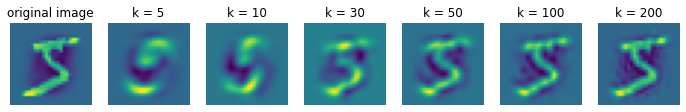Starting from $k = 50$, we see the digit 5 clearly. What does this mean? The original picture is of size $28 \times 28$, so we need 784 numbers to represent such an image. But with PCA, we can use only $50$ numbers to represent the same image with losing the digits. This is a common way we reduce the number of features in machine learning, especially in deep learning.

Linear Model with PCA. In the lecture on Linear Regression, we use a linear model to regconize handwritten digits with an accuracy about 86%. The linear model used is a map from $\mathbb{R}^{784}$ to $\mathbb{R}^{10}$ with 7850 parameters. We can use PCA to reduce the number of parameters dramatically while maintaining the same level accuracy. In the followings, we will use the first $k = 50$ components.

k = 50
x = normalized_mnist_images @ mnist_vectors[:, range(k)]
y = np.zeros((mnist_labels.size, 10))
y[np.arange(mnist_labels.size), mnist_labels] = 1
model = sklearn.linear_model.LinearRegression().fit(x, y)
print(f'''If we write the linear model as T(x) = Ax + B, then:
A is of the shape: {model.coef_.shape}
B is of the shape: {model.intercept_.shape}
Total number of parameters: {11 * k}
''')

If we write the linear model as T(x) = Ax + B, then:
A is of the shape: (10, 50)
B is of the shape: (10,)
Total number of parameters: 550


predicted_labels = np.argmax(model.predict(x), axis=1)
train_accuracy = np.sum(predicted_labels == mnist_labels) / mnist_labels.size
print(f"Train accuracy: {train_accuracy * 100:.2f}%")

Train accuracy: 84.02%


Since we can represent a picture using 50 components, so the total number of parameters needed in the linear model is only 550. Compared to the original 7850 parameters with 86% accuracy, 550 parameters with 84% accuracy is very good. This sort of parameters reduction is more important if we have a deep neural network.

## Eigenfaces

If we apply PCA to a dataset of faces, we call the obtained eigenvectors eigenfaces of the dataset. We take Yale Face Database (http://vision.ucsd.edu/content/yale-face-database) as an exmple.

gz = tarfile.open("yalefaces.tar.gz", "r:gz")
confs = [
'centerlight', 'glasses', 'happy', 'leftlight',
'noglasses', 'normal', 'rightlight', 'sad',
'sleepy', 'surprised', 'wink'
]
faces = {
c: [] for c in confs
}
for sj in range(1, 16):
for c in confs:
name = f"./yalefaces/subject{sj:02}.{c}"
faces[c].append(Image.open(gz.extractfile(name)))


The dataset contains 15 subjects, and 11 configurations for each subjects. Here is the list of faces with different configurations for subject 1.

plt.figure(figsize=(12, 3))
n = len(confs)
for i in range(n):
plt.subplot(1, n, i + 1)
plt.imshow(faces[confs[i]], cmap="gray")
plt.title(confs[i])
plt.axis("off")
plt.show()width, height = faces['centerlight'].size
vecsize = height * width
memsize = vecsize * vecsize * 8 / 1e9
print(f'''The image size of each face is ({height}, {width}),
so it is a vector of size {vecsize}.
The covariance matrix is of size {vecsize} x {vecsize}.
If each number takes 8 bytes, then this matrix takes {memsize:.2f} GB.''')

The image size of each face is (243, 320),
so it is a vector of size 77760.
The covariance matrix is of size 77760 x 77760.
If each number takes 8 bytes, then this matrix takes 48.37 GB.


The covariance matrix is too large to fit in the RAM of a regular computer or labtop. We must need a better algorithm to find principal components from a large data. Here is a simple trick from the observation that we has much smaller number of rows than that of columns.

Proposition. Let $X = A^T A$ and $Y = AA^T$, where $A^T$ is the transpose of $A$. Suppose $v$ is an eigenvector of $Y$ corresponding to the eigenvalue $\lambda$ of $Y$. Then $A^T v$ is an eigenvector of $X$ corresponding to the eigenvalue $\lambda$ of $X$, as long as $A^Tv$ is not zero.

Proof. We have

$$X(A^Tv) = A^TAA^Tv = A^T (Yv) = \lambda A^T v.$$

Thus, if $A^Tv$ is not zero, then it is a $\lambda$-eigenvector of $X$.

Suppose our faces data are stored in $A$ with each row representing one face, so $A$ is of size $165 \times 77760$. We want to find eigenvectors of $X = A^TA$, which is a large $77760 \times 77760$ matrix. By the proposition, we can change the problem to finding eigenvectors of $Y = AA^T$, which is a much smaller $165 \times 165$ matrix.

faces_data = []
for val in faces.values():
for img in val:
faces_data.append(np.array(img).flatten())
faces_mat = np.array(faces_data)
faces_mean = np.mean(faces_mat, axis=0, keepdims=True)

# the matrix A = faces_mat_normalized
faces_mat_normalized = faces_mat - faces_mean

# Y = AA^T
Y = faces_mat_normalized @ faces_mat_normalized.transpose()
# find eigenvector v of Y
# Y is symmetric, its eigenvalues are real
eigenvalues, eigenvectors = map(np.real, np.linalg.eig(Y))
# A^T v is the eigenvector of X = A^TA
eigenfaces = faces_mat_normalized.transpose() @ eigenvectors

# sort by eigenvalues in descending order
idx = np.argsort(eigenvalues)[::-1]
eigenvalues = eigenvalues[idx]
eigenfaces = eigenfaces[:, idx]

# normalize each eigenface to length 1
eigenfaces_norm = np.linalg.norm(eigenfaces, axis=0, keepdims=True)
eigenfaces /= eigenfaces_norm

# transpose to make each row to represent an eigenface
eigenfaces = eigenfaces.transpose()
eigenfaces.shape

(165, 77760)

Each row is an eigenface. We only get 165 eigenfaces, since we use the proposition above to find eigenvectors of a much smaller matrix. However, we don't have anything to lose, becuase there are only 165 faces in the dataset, so we can surely embed our dataset in $\mathbb{R}^{165}$.

We now look at the first 6 eigenfaces of the dataset.

r, c = 3, 2
fig, ax = plt.subplots(r, c)
for i in range(r):
for j in range(c):
k = i * c + j
img = eigenfaces[k]
img = img.reshape((height, width))
im = ax[i, j].imshow(img)
fig.colorbar(im, ax=ax[i, j])
ax[i, j].set_title(f"eigenface {k + 1}")
ax[i, j].axis('off')
plt.show()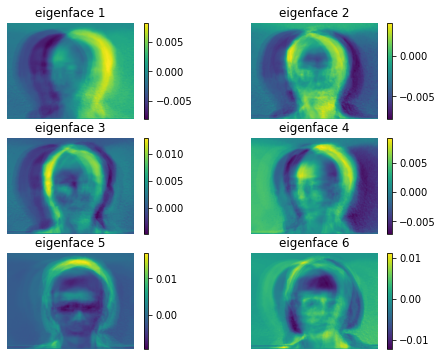We see a lot of ghost faces in these eigenfaces. This is good, because it tells us where to find a face in an image. Each eigenface includes other features other than faces as well. For example, to the right of the yellow-ish face in the middle of eigenface 2, the dark purple shape is trying to capture hair in an image.

Next, we compute components of all faces with respect to these 165 eigenfaces. For each face, we should have 165 compontents.

# Normalization to zero mean
faces_mat_normalized = faces_mat - faces_mean

# Compute components of all faces
# Each row is the set of components for a face
faces_components = faces_mat_normalized @ eigenfaces.T


PCA can help us visualize high dimension data. For example, we can represent each high dimensional point using only two principal components, for which we can draw on a 2D plane. In our case, each face is of 77760 dimension. Let's project all faces onto the first two principal components plane.

# We group each subject together
colors = [
"red", "chocolate", "darkorange", "gold", "olive",
"yellowgreen", "chartreuse", "forestgreen", "aqua", "dodgerblue",
"navy", "slateblue", "hotpink", "darkviolet", "violet"
]
for sj in range(15):
# get first components for all configurations of a subject
x = faces_components[sj::15, 0]

# get second components for all configurations of a subject
y = faces_components[sj::15, 1]

plt.plot(x, y, 'o', color=colors[sj], label=f'subject {sj + 1}')

plt.xlabel("first component")
plt.ylabel("second component")
plt.title("Visualization of faces in 2D")
plt.legend(bbox_to_anchor=(1, 1))
plt.show()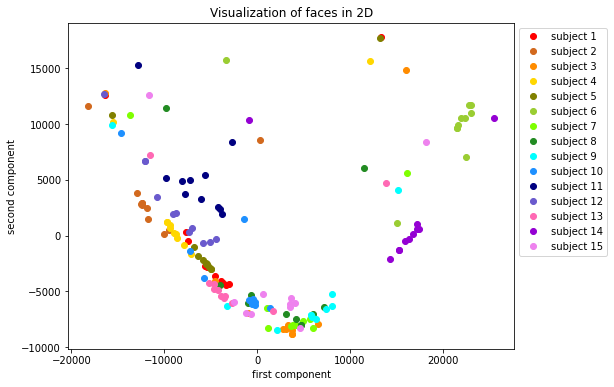We group each subject with different configurations together. Each group is more or less in its own cluster, and somewhat distinguishable from other subjects. Thus, PCA and cosine similarity should be able to separate each subject. We would like to see each configuration as a group as well. Below is the code to do so.

for c in range(11):
# get first components for all configurations of a subject
x = faces_components[c * 15: (c + 1) * 15, 0]

# get second components for all configurations of a subject
y = faces_components[c * 15: (c + 1) * 15, 1]

plt.plot(x, y, 'o', color=colors[c], label=f'{confs[c]}')

plt.xlabel("first component")
plt.ylabel("second component")
plt.title("Visualization of configurations in 2D")
plt.legend(bbox_to_anchor=(1, 1))
plt.show()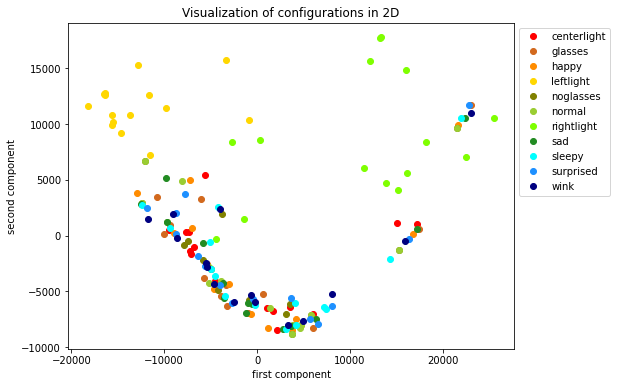We see that only leftlight and rightlight configurations are obviously away from others in this 2D visualization.

We now want to see how many eigenfaces do we need to reconstruct a facial image in the dataset. We project 3 selected images into subspaces spanned by different numbers of eigenfaces.

cur_idx = 1
num_of_components = [10, 30, 50, 100, 150, 165]
n = len(num_of_components)
for origin_img in faces_mat[[1, 41, 70]]:
img = origin_img - faces_mean

plt.figure(figsize=(12, 3))
plt.subplot(3, n + 1, cur_idx)
cur_idx += 1
plt.imshow(img.reshape((height, width)), cmap="gray")
plt.axis('off')
if cur_idx < n:
plt.title("original")

components = [
np.dot(img, eigenfaces[i])
for i in range(num_of_components[-1])
]
for j, k in enumerate(num_of_components):
plt.subplot(3, n + 1, cur_idx)
cur_idx += 1
img_proj = sum(components[i] * eigenfaces[i] for i in range(k))
plt.imshow(img_proj.reshape((height, width)), cmap="gray")
plt.axis("off")
if cur_idx < n + 3:
plt.title(f"k = {k}")

plt.show()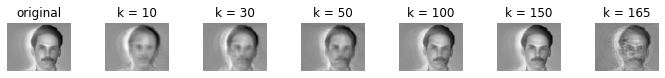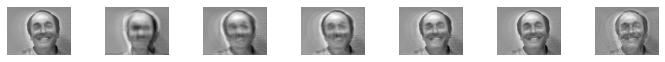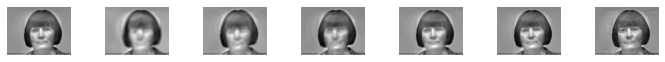The constructed images are in better quality as $k$ grows upto 150, but seem worse when $k$ is 165. But why? Shouldn't it be better with more components? To answer this, we can compuate the discrepency between the constructed images and original images. For each image $u$, let $u_k$ be the projection of $u$ onto the first $k$ principal components. We use the square of distance to quantify the discrepency:

$$|u - u_k|^2.$$

The the total error for all faces using $k$ components is

$$E(k) = \sum_{u} |u - u_k|^2.$$

We will compute all $E(k)$ for $1 \le k \le 165$ and plot them.

@np.vectorize
def E(k):
projs = faces_components[:,range(k)] @ eigenfaces[range(k), :]
diff = projs - faces_mat_normalized
return np.sum(diff ** 2)

component_nums = np.arange(1, 166)
errors = E(component_nums)
idx = np.argmin(errors)
num = idx + 1

plt.plot(component_nums, errors)
plt.annotate(
f"error minimized at {num} components",
xy=(idx, errors[idx]),
xytext=(75, 1e10),
arrowprops=dict(
color='red',
width=1
)
)
plt.xlabel("k")
plt.ylabel("E")
plt.title("errors vs component numbers")
plt.show()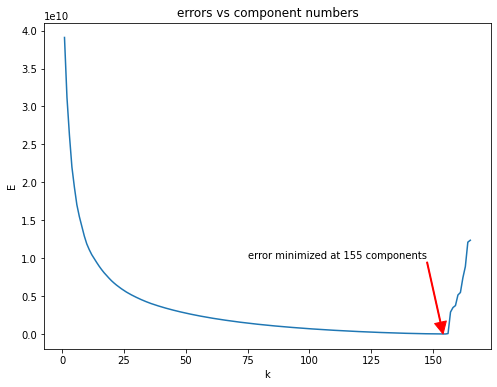We confirm from the above figure that the errors go up after 155 components. The reason is that the our face data matrix $A$, which is stored in faces_mat_normalized, is of rank 155.

print(f'''The rank of A = faces_mat_normalized: {np.linalg.matrix_rank(faces_mat_normalized)}.
The rank of Y = AA^T: {np.linalg.matrix_rank(Y)}.

The last 15 eigenvalues of Y (and thus X):
{eigenvalues[-15:]}
''')

The rank of A = faces_mat_normalized: 155.
The rank of Y = AA^T: 155.

The last 15 eigenvalues of Y (and thus X):
[ 4.66840183e+06  3.17103757e+06  2.92078690e+06  1.50538690e+06
1.26715146e+06  5.41898694e-08  1.10068611e-08  9.04219354e-09
2.97125122e-09  2.97125122e-09 -1.38617835e-09 -2.74487910e-09
-9.17813263e-09 -9.17813263e-09 -9.25369756e-08]



Thus, we should have eigenvalues 0 appearing 10 times. As a matter of fact, if we look at the last 15 eigenvalues, we can see that the last 10 are close to 0 while others are much much bigger than 0. (We don't have them exactly 0 because of the numerical errors in numpy.) Therefore, the last 10 components will not make the reconstructed faces look better, but only add noises to them.

After the discussion, we take $k=155$ principal components to represent faces. That means, to represent a face now only requires 155 numbers, while originally requires 77760 numbers. We save 99.8% of the memory by applying PCA!